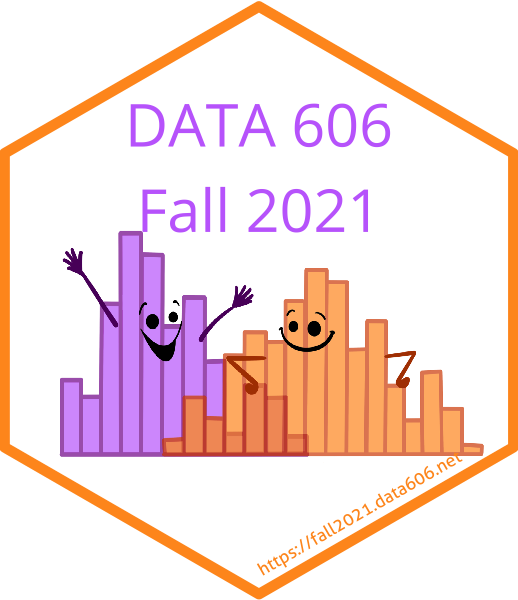# Chapter 9

## Multiple and Logistic Regression

### Learning Outcomes

• Define the multiple linear regression model as $$\hat{y} = \beta_0 + \beta_1 x_1 + \beta_2 x_2 + \cdots + \beta_k x_k$$ where there are $k$ predictors (explanatory variables).
• Interpret the estimate for the intercept ($b_0$) as the expected value of $y$ when all predictors are equal to 0, on average.
• Interpret the estimate for a slope (say $b_1$) as “All else held constant, for each unit increase in $x_1$, we would expect $y$ to increase/decrease on average by $b_1$.”
• Define collinearity as a high correlation between two independent variables such that the two variables contribute redundant information to the model – which is something we want to avoid in multiple linear regression.
• Note that $R^2$ will increase with each explanatory variable added to the model, regardless of whether or not the added variables is a meaningful predictor of the response variable. Therefore we use adjusted $R^2$, which applies a penalty for the number of predictors included in the model, to better assess the strength of a multiple linear regression model: $$R^2 = 1 - \frac{Var(e_i) / (n - k - 1)}{Var(y_i) / (n - 1)}$$ where $Var(e_i)$ measures the variability of residuals ($SS_{Err}$), $Var(y_i)$ measures the total variability in observed $y$ ($SS_{Tot}$), $n$ is the number of cases and $k$ is the number of predictors.
• Note that adjusted $R^2$ will only increase if the added variable has a meaningful contribution to the amount of explained variability in $y$, i.e. if the gains from adding the variable exceeds the penalty.
• Define model selection as identifying the best model for predicting a given response variable.
• Note that we usually prefer simpler (parsimonious) models over more complicated ones.
• Define the full model as the model with all explanatory variables included as predictors.
• Note that the p-values associated with each predictor are conditional on other variables being included in the model, so they can be used to assess if a given predictor is significant, given that all others are in the model.
• These p-values are calculated based on a $t$ distribution with $n - k - 1$ degrees of freedom.
• The same degrees of freedom can be used to construct a confidence interval for the slope parameter of each predictor: $$b_i \pm t^\star_{n - k - 1} SE_{b_i}$$
• Stepwise model selection (backward or forward) can be done based based on adjusted $R^2$ (choose the model with higher adjusted $R^2$).
• The general idea behind backward-selection is to start with the full model and eliminate one variable at a time until the ideal model is reached. i. Start with the full model. ii. Refit all possible models omitting one variable at a time, and choose the model with the highest adjusted $R^2$. iii. Repeat until maximum possible adjusted $R^2$ is reached.
• The general idea behind forward-selection is to start with only one variable and adding one variable at a time until the ideal model is reached. i. Try all possible simple linear regression models predicting $y$ using one explanatory variable at a time. Choose the model with the highest adjusted $R^2$. ii. Try all possible models adding one more explanatory variable at a time, and choose the model with the highest adjusted $R^2$. iii. Repeat until maximum possible adjusted $R^2$ is reached.
• Adjusted $R^2$ method is more computationally intensive, but it is more reliable, since it doesn’t depend on an arbitrary significant level.
• List the conditions for multiple linear regression as
1. linear relationship between each (numerical) explanatory variable and the response - checked using scatterplots of $y$ vs. each $x$, and residuals plots of $residuals$ vs. each $x$
2. nearly normal residuals with mean 0 - checked using a normal probability plot and histogram of residuals
3. constant variability of residuals - checked using residuals plots of $residuals$ vs. $\hat{y}$, and $residuals$ vs. each $x$
4. independence of residuals (and hence observations) - checked using a scatterplot of $residuals$ vs. order of data collection (will reveal non-independence if data have time series structure)
• Note that no model is perfect, but even imperfect models can be useful.